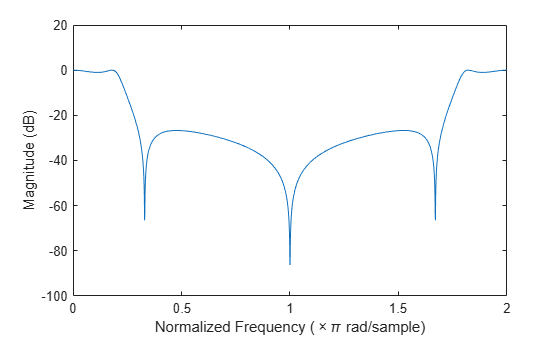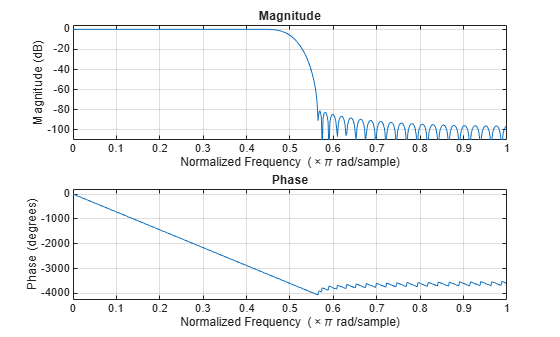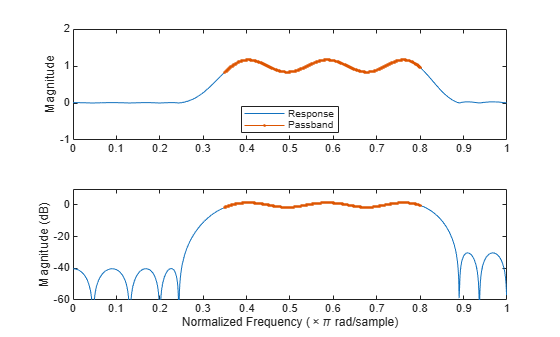# freqz

Frequency response of digital filter

## Syntax

[h,w] = freqz(b,a,n)
[h,w] = freqz(sos,n)
[h,w] = freqz(d,n)
[h,w] = freqz(___,n,'whole')
[h,f] = freqz(___,n,fs)
[h,f] = freqz(___,n,'whole',fs)
h = freqz(___,w)
h = freqz(___,f,fs)
freqz(___)

## Description

example

[h,w] = freqz(b,a,n) returns the n-point frequency response vector h and the corresponding angular frequency vector w for the digital filter with transfer function coefficients stored in b and a.

example

[h,w] = freqz(sos,n) returns the n-point complex frequency response corresponding to the second-order sections matrix sos.

example

[h,w] = freqz(d,n) returns the n-point complex frequency response for the digital filter d.
[h,w] = freqz(___,n,'whole') returns the frequency response at n sample points around the entire unit circle.
[h,f] = freqz(___,n,fs) returns the frequency response vector h and the corresponding physical frequency vector f for a digital filter designed to filter signals sampled at a rate fs.
[h,f] = freqz(___,n,'whole',fs) returns the frequency vector at n points ranging between 0 and fs.
h = freqz(___,w) returns the frequency response vector h evaluated at the normalized frequencies supplied in w.
h = freqz(___,f,fs) returns the frequency response vector h evaluated at the physical frequencies supplied in f.

example

freqz(___) with no output arguments plots the frequency response of the filter.

## Examples

collapse all

Compute and display the magnitude response of the third-order IIR lowpass filter described by the following transfer function:

$H\left(z\right)=\frac{0.05634\left(1+{z}^{-1}\right)\left(1-1.0166{z}^{-1}+{z}^{-2}\right)}{\left(1-0.683{z}^{-1}\right)\left(1-1.4461{z}^{-1}+0.7957{z}^{-2}\right)}.$

Express the numerator and denominator as polynomial convolutions. Find the frequency response at 2001 points spanning the complete unit circle.

b0 = 0.05634; b1 = [1 1]; b2 = [1 -1.0166 1]; a1 = [1 -0.683]; a2 = [1 -1.4461 0.7957]; b = b0*conv(b1,b2); a = conv(a1,a2); [h,w] = freqz(b,a,'whole',2001);

Plot the magnitude response expressed in decibels.

plot(w/pi,20*log10(abs(h))) ax = gca; ax.YLim = [-100 20]; ax.XTick = 0:.5:2; xlabel('Normalized Frequency (\times\pi rad/sample)') ylabel('Magnitude (dB)')Compute and display the magnitude response of the third-order IIR lowpass filter described by the following transfer function:

$H\left(z\right)=\frac{0.05634\left(1+{z}^{-1}\right)\left(1-1.0166{z}^{-1}+{z}^{-2}\right)}{\left(1-0.683{z}^{-1}\right)\left(1-1.4461{z}^{-1}+0.7957{z}^{-2}\right)}.$

Express the transfer function in terms of second-order sections. Find the frequency response at 2001 points spanning the complete unit circle.

b0 = 0.05634; b1 = [1 1]; b2 = [1 -1.0166 1]; a1 = [1 -0.683]; a2 = [1 -1.4461 0.7957]; sos1 = [b0*[b1 0] [a1 0]]; sos2 = [b2 a2]; [h,w] = freqz([sos1;sos2],'whole',2001);

Plot the magnitude response expressed in decibels.

plot(w/pi,20*log10(abs(h))) ax = gca; ax.YLim = [-100 20]; ax.XTick = 0:.5:2; xlabel('Normalized Frequency (\times\pi rad/sample)') ylabel('Magnitude (dB)')Design an FIR lowpass filter of order 80 using a Kaiser window with $\beta =8$. Specify a normalized cutoff frequency of $0.5\pi$ rad/sample. Display the magnitude and phase responses of the filter.

b = fir1(80,0.5,kaiser(81,8)); freqz(b,1)Design the same filter using designfilt. Display its magnitude and phase responses using fvtool.

d = designfilt('lowpassfir','FilterOrder',80, ... 'CutoffFrequency',0.5,'Window',{'kaiser',8}); freqz(d)Design an FIR bandpass filter with passband between $0.35\pi$ and $0.8\pi$ rad/sample and 3 dB of ripple. The first stopband goes from $0$ to $0.1\pi$ rad/sample and has an attenuation of 40 dB. The second stopband goes from $0.9\pi$ rad/sample to the Nyquist frequency and has an attenuation of 30 dB. Compute the frequency response. Plot its magnitude in both linear units and decibels. Highlight the passband.

sf1 = 0.1; pf1 = 0.35; pf2 = 0.8; sf2 = 0.9; pb = linspace(pf1,pf2,1e3)*pi; bp = designfilt('bandpassfir', ... 'StopbandAttenuation1',40, 'StopbandFrequency1',sf1,... 'PassbandFrequency1',pf1,'PassbandRipple',3,'PassbandFrequency2',pf2, ... 'StopbandFrequency2',sf2,'StopbandAttenuation2',30); [h,w] = freqz(bp,1024); hpb = freqz(bp,pb); subplot(2,1,1) plot(w/pi,abs(h),pb/pi,abs(hpb),'.-') axis([0 1 -1 2]) legend('Response','Passband','Location','South') ylabel('Magnitude') subplot(2,1,2) plot(w/pi,db(h),pb/pi,db(hpb),'.-') axis([0 1 -60 10]) xlabel('Normalized Frequency (\times\pi rad/sample)') ylabel('Magnitude (dB)')## Input Arguments

collapse all

Transfer function coefficients, specified as vectors. Express the transfer function in terms of b and a as

$H\left({e}^{j\omega }\right)=\frac{B\left({e}^{j\omega }\right)}{A\left({e}^{j\omega }\right)}=\frac{\text{b(1)}+\text{b(2)}\text{\hspace{0.17em}}{e}^{-j\omega }+\text{b(3)}\text{\hspace{0.17em}}{e}^{-j2\omega }+\cdots +\text{b(M)}\text{\hspace{0.17em}}{e}^{-j\left(M-1\right)\omega }}{\text{a(1)}+\text{a(2)}\text{\hspace{0.17em}}{e}^{-j\omega }+\text{a(3)}\text{\hspace{0.17em}}{e}^{-j2\omega }+\cdots +\text{a(N)}\text{\hspace{0.17em}}{e}^{-j\left(N-1\right)\omega }}.$

Example: b = [1 3 3 1]/6 and a = [3 0 1 0]/3 specify a third-order Butterworth filter with normalized 3 dB frequency 0.5π rad/sample.

Data Types: double | single
Complex Number Support: Yes

Number of evaluation points, specified as a positive integer scalar no less than 2. When n is absent, it defaults to 512. For best results, set n to a value greater than the filter order.

Second-order section coefficients, specified as a matrix. sos is a K-by-6 matrix, where the number of sections, K, must be greater than or equal to 2. If the number of sections is less than 2, the function treats the input as a numerator vector. Each row of sos corresponds to the coefficients of a second-order (biquad) filter. The ith row of sos corresponds to [bi(1) bi(2) bi(3) ai(1) ai(2) ai(3)].

Example: s = [2 4 2 6 0 2;3 3 0 6 0 0] specifies a third-order Butterworth filter with normalized 3 dB frequency 0.5π rad/sample.

Data Types: double | single
Complex Number Support: Yes

Digital filter, specified as a digitalFilter object. Use designfilt to generate a digital filter based on frequency-response specifications.

Example: d = designfilt('lowpassiir','FilterOrder',3,'HalfPowerFrequency',0.5) specifies a third-order Butterworth filter with normalized 3 dB frequency 0.5π rad/sample.

Sample rate, specified as a positive scalar. When the unit of time is seconds, fs is expressed in hertz.

Data Types: double

Angular frequencies, specified as a vector and expressed in rad/sample. w must have at least two elements, because otherwise the function interprets it as n. w = π corresponds to the Nyquist frequency.

Frequencies, specified as a vector. f must have at least two elements, because otherwise the function interprets it as n. When the unit of time is seconds, f is expressed in hertz.

Data Types: double

## Output Arguments

collapse all

Frequency response, returned as a vector. If you specify n, then h has length n. If you do not specify n, or specify n as the empty vector, then h has length 512.

If the input to freqz is single precision, the function computes the frequency response using single-precision arithmetic. The output h is single precision.

Angular frequencies, returned as a vector. w has values ranging from 0 to π. If you specify 'whole' in your input, the values in w range from 0 to 2π. If you specify n, w has length n. If you do not specify n, or specify n as the empty vector, w has length 512.

Frequencies, returned as a vector expressed in hertz. f has values ranging from 0 to fs/2 Hz. If you specify 'whole' in your input, the values in f range from 0 to fs Hz. If you specify n, f has length n. If you do not specify n, or specify n as the empty vector, f has length 512.

## Algorithms

The frequency response of a digital filter can be interpreted as the transfer function evaluated at z = e .

freqz determines the transfer function from the (real or complex) numerator and denominator polynomials you specify and returns the complex frequency response, H(e), of a digital filter. The frequency response is evaluated at sample points determined by the syntax that you use.

freqz generally uses an FFT algorithm to compute the frequency response whenever you do not supply a vector of frequencies as an input argument. It computes the frequency response as the ratio of the transformed numerator and denominator coefficients, padded with zeros to the desired length.

When you do supply a vector of frequencies as input, freqz evaluates the polynomials at each frequency point and divides the numerator response by the denominator response. To evaluate the polynomials, the function uses Horner’s method.

 Oppenheim, Alan V., Ronald W. Schafer, and John R. Buck. Discrete-Time Signal Processing. 2nd Ed. Upper Saddle River, NJ: Prentice Hall, 1999.